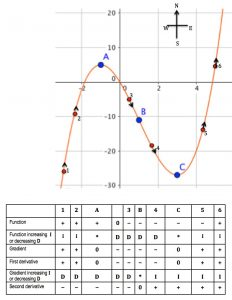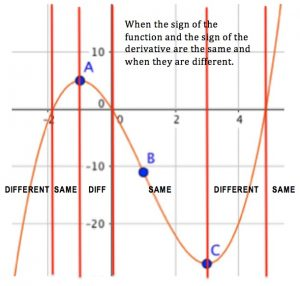Imagine this graph is a road and you are driving from the bottom left hand (South West) corner to the top right hand (North East) corner. Describe how you would steer the car.

When would you be turning the steering wheel to the right?

When would you be turning the steering wheel to the left?

When would you be driving straight ahead?

Now look at the graph again. What was happening to the gradient when you were steering to the right? What was happening to the gradient when you were steering to the left. What was happening to the gradient when you were steering straight ahead? What was the gradient when you were travelling due East?

This is the graph of $f(x) = x^3 - 3x^2 - 9x$.

Find the first derivative and the second derivative of this function and the coordinates of points A, B and C.When do this cubic function and its first derivative have the same sign?

Sketch the graphs of a straight line and of a quadratic function and describe when these functions and their first derivatives have the same sign and when they have different signs.

Tagged with: